## research papersJOURNAL OFSYNCHROTRONRADIATION
ISSN: 1600-5775
Volume 15| Part 5| September 2008| Pages 458-462

## The optimum conditions to collect X-ray data from very small samples

aSTFC Daresbury Laboratory, Daresbury, Warrington WA4 4AD, UK, and bDiamond Light Source Ltd, Diamond House, Harwell Science and Innovation Campus, Didcot, Oxfordshire OX11 0DE, UK
*Correspondence e-mail: colin.nave@diamond.ac.uk

(Received 5 February 2008; accepted 14 May 2008; online 22 July 2008)

A previous paper [Nave & Hill (2005). J. Synchrotron Rad. 12, 299–303] examined the possibility of reduced radiation damage for small crystals (10 µm and below in size) under conditions where the photoelectrons could escape from the sample. The conclusion of this paper was that higher-energy radiation (e.g. 40 keV) could offer an advantage as the photoelectron path length was greater and less energy would be deposited in the crystal. This paper refines these calculations further by including the effects of energy deposited owing to Compton scattering and the energy difference between the incident photon and the emitted photoelectron. An estimate is given for the optimum wavelength for collecting data from a protein crystal of a given size and composition. Another way of reducing radiation damage from a protein crystal is to collect data with a very short pulsed X-ray source where a single image can be obtained before subsequent radiation damage occurs. A comparison of this approach compared with the use of shorter wavelengths is made.

### 1. Introduction

There is an increasing awareness of the possibility of radiation damage during X-ray data collection from protein crystals at cryotemperatures and it is possible to fold this factor into the data collection strategy (Popov & Bourenkov, 2003). In addition, there is the possibility of actually exploiting the radiation damage for phasing (Ravelli et al., 2003). However, minimizing the damage in the first place will offer significant benefits, particularly for small crystals where a high dose has to be given in order to collect the necessary data. The use of scavengers is one possible approach to reducing the damage, and developments are underway in this area (Murray & Garman, 2002; Southworth-Davies & Garman, 2007; Kauffman et al., 2006). This approach requires altering the conditions in the crystal and may not be applicable for all samples. A complementary approach (which can be used combined with or instead of scavengers) is to ensure that the properties of the X-ray source are optimized for the particular sample and the detector is also matched. Parameters such as the size and divergence of the X-ray beam should be matched to the sample properties in a way which ensures an even distribution of dose in the sample, minimization of background and preservation of small spot sizes compatible with the detector resolution. Some of the issues related to this are discussed by Nave (1998, 1999).

In addition to the spatial dimensions and divergence of the beam, the energy and temporal nature are also relevant. This paper is mainly concerned with calculations to find the optimum energy of the incident beam to minimize the ratio between the energy deposited in the sample and the intensity of the scattered photons used for structure determination. A comparison is also made with the use of ultra-short pulses of radiation to collect diffraction data before radiation damage occurs.

The main issue is to maximize the number of diffraction patterns which could be obtained from a specific crystal. As a baseline, one can use a rule of thumb' developed by Glaeser et al. (2000). This stated that one high-resolution diffraction pattern, 1° of rotation, can be obtained from a protein crystal if the crystal size (in micrometres) is one-tenth of the unit-cell size (in Å). This is a useful way of addressing the issue as it includes both crystal size and unit-cell dimension. In practise, different crystals might be more or less tolerant to radiation damage owing to, for example, very low or high solvent content. Coulibaly et al. (2007) showed that entire 2 Å data sets could be collected from 5–12 µm crystals with unit-cell dimensions of 103 Å and a solvent content of 19%. In this paper we factor out parameters such as the contents of the unit cell, the size of the crystal, the size of the unit cell and the resolution. This is done by defining a reduction in the dose required to obtain a particular set of diffraction data when effects such as photoelectron escape are not present. The reduction is taken to be unity at 12 keV (the wavelength considered by Glaeser et al., 2000).

### 2. Method of calculation

The various parameters of interest are as follows.

D(E): relative dose required (scaled to unity at E = 12 keV) assuming energy deposition by photoelectric absorption but no photoelectron escape.

DC(E): dose required as above but including energy deposition due to Compton scattering.

DCE(E): dose required as above (DC) taking into account photoelectron escape.

E: energy of incident photon.

λ: wavelength of incident photon.

EPE: photoelectron energy.

EK: K-shell energy.

EC: average energy of Compton electron.

EAPE: average energy remaining in the crystal after photoelectron escape.

σPE: photoelectric cross section.

σC: Compton cross section.

The deposited energy (dose absorbed) compared with the number of photons in a diffraction spot is the quantity one would like to minimize. There is a dependence on photon energy for this ratio for the case where no Compton scattering occurs, and the photoelectrons remain entirely within the crystal. When this is the case, the absorption coefficient for the protein needs to be multiplied by the energy to take account of the increased energy deposited for a higher-energy photon absorbed. The integrated intensity in a diffraction spot for a mosaic crystal, ignoring polarization effects, is proportional to λ3/sin(2θ), where θ is the Bragg angle (see, for example, International Tables for Crystallography, Vol. C, Table 6.2.1.1, pp. 597–598). For small Bragg angles this is approximately proportional to λ2. The dose absorbed, scaled to the integrated intensity of a diffraction spot, is therefore given by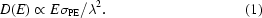Inclusion of Compton scattering gives the modified formula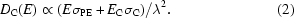Cross sections for Compton scattering and photoelectric absorption were obtained from (https://physics.nist.gov/PhysRefData/Xcom/html/xcom1.html ). The maximum energy of the Compton electron can be calculated from the Compton shift formula [see, for example, International Tables for Crystallography, Vol. C, equation (7.4.3.1), pp. 657] at a scattering angle of 180° which is 2E2/mc2[1 + 2E/mc2], where mc2 corresponds to the electron rest energy of 511 keV. This value was divided by two for the average Compton energy. For an example of these calculations, see International Tables for Crystallography, Vol. C, Table 7.4.3.1, pp. 657.

For the case of small crystals, the term EPσPE needs to be modified to allow for the escape of the photoelectrons from the crystal. The average photoelectron energy which remains in the crystal (EAPE) is calculated using the program Casino (Hovington et al., 1997) as described by Nave & Hill (2005). The photoelectron could be created anywhere in the parts of the crystal illuminated with X-rays and be emitted in a direction requiring it to travel the total thickness of the crystal before it escaped. This was handled by dividing the crystal up into five slabs and carrying out the calculation for each one. For example, in a crystal of thickness 10 µm, EAPE was calculated for electrons produced 1, 3, 5, 7 and 9 µm away from the surface and the total energy was calculated from a weighted sum. For each energy and each width of sample, a simulation of 105 electrons in a protein crystal of composition C1818H7286N420O2673S25 with a density of 1.17 g cm−3 was run as described by Nave & Hill (2005). For the case considered here, where the primary photoelectric absorption events take place in the K-shell, an additional term EK should be added to allow for the difference between the energy of the photoelectron and that of the photon, giving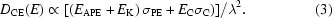Values for EAPE were obtained for photoelectron energies between 1 and 40 keV in 1 keV intervals. Values at a particular photon energy were obtained by linear interpolation between these values. Some approximations and assumptions are made in these calculations and these are discussed below, with an estimate of the error which is present when the appropriate factors are ignored.

(i) The assumption (implicit in the program Casino) is that any photoelectrons which escape do so from a face in the forward-scattered direction (with respect to the initial direction of the photoelectron) and that any back-scattered or side-scattered electrons will remain within the crystal. Some of these electrons could escape if an alternative nearby crystal face was present, leading to an underestimate of the energy which escapes the crystal. Fig. 1shows an example for 17 keV photoelectrons generated 0.75 µm from a surface in the forward direction. Of the 200 electron tracks shown on the diagram, three are back-scattered and three are side-scattered. Only part of the initial energy of these photoelectrons would escape the crystal. The approximation made by assuming all the energy remains in the crystal would therefore lead to an underestimation of transmitted energy of less than 3%. In any case, the proportion of back- and side-scattered electrons decreases for the cases of most interest here, where the energy of the photoelectrons is greater.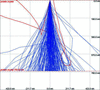Figure 1 Tracks generated 0.75 µm from the surface of a protein crystal for photoelectrons of 17.5 keV as calculated by the program Casino.

(ii) The difference between the photoelectron energy and the photon energy results in either the production of X-ray fluorescence or Auger electrons. It is assumed that these, with their associated energy, remain in the crystal. This is a reasonable assumption as Auger electrons and the fluorescent X-rays from light atoms considered here have a low energy. However, if heavier atoms were present (e.g. Se, Hg, Br), then the fluorescence yield and the energy of the fluorescent X-ray (together with its likelihood of escape) will have to be taken into account.

(iii) The numerical simulation was carried out by assuming that the photoelectron was created at different distances from the surface of the crystal using five points at different depths throughout the crystal. The linear distribution of depths was chosen for simplicity in the calculations. This leads to small discontinuities in the graphs of DCE plotted against energy, affecting the less relevant values of DCE above 0.8 (see Fig. 3).

(iv) Various parameters related to the specific diffraction geometry are not included. The approximation sin(2θ) ∝ λ for the Lorentz factor is made. This latter approximation could only be avoided if a specific Bragg resolution (d-spacing) was selected for the analysis.

Owing to the approximations in a real experiment, not least estimating the thickness of real crystals with sufficient accuracy, it is not considered useful to make more accurate simulations than used here.

### 3. Results of calculations

Fig. 2shows the ratio of dose absorbed to scattered intensity in a diffraction spot assuming no escape of photoelectrons, in the absence of Compton scattering [equation (1)] and with Compton scattering [equation (2)]. This ratio varies by less than 40% over the energy range 1–40 keV. The variation is due to the fact that the cross sections for photoelectric absorption do not vary precisely as λ3 over this energy range. The reduction in radiation damage owing to the escape of photoelectrons [equation (3)] is shown for crystals of various sizes in Fig. 3.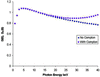Figure 2 Ratio of dose to scatter in a protein crystal scaled to the value at 12 keV with and without the inclusion of Compton scattering.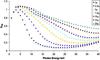Figure 3 Ratio of dose to scatter (as in Fig. 1) for crystals of different sizes, including the effect of Compton scattering and photoelectron escape.

### 4. Discussion

The reduction in dose which could be obtained should be used for guidance only. For example, if one could only obtain one diffraction pattern from a 2.5 µm crystal, 25 Å cell at 12 keV, increasing the X-ray energy to 25 keV would allow seven diffraction patterns to be obtained. Some of the minima in the curves in Fig. 3are quite broad, so a significant gain could be obtained for a wide range of crystal sizes if operating in the 20–30 keV range. The main conclusion of this paper is that there should be a significant advantage in collecting data at these energies for small crystals provided other factors (e.g. detector efficiency) remain optimized.

Since Nave & Hill (2005), several authors have suggested that the radiation damage effects which they see may have been reduced by photoelectron escape (Beitlich et al., 2007; Boutet & Robinson, 2006; Riekel et al., 2005). However, these have not generally been controlled experiments specifically designed to investigate these effects. Such experiments could be carried out in a number of ways, where possible exploiting the fact that the photoelectrons are emitted around the direction of polarization of the X-ray beam. Crystals of varying size could be examined at a fixed X-ray energy in order to see if the decay of diffraction intensities was reduced for the smaller crystals. With a suitable geometry, X-ray beams of a few micrometres in size could be used with larger crystals and the decay compared with use of a larger X-ray beam, for which many of the photoelectrons will remain in the exposed volume. A recent paper (Moukhametzianov et al., 2008) described this approach. Results of simulations (ignoring Compton scattering and retention of energy in the K-shell) were included in this paper at energies up to 15.7 keV. The conclusion was that the reduced radiation damage observed was consistent with photoelectron escape. Finally, and perhaps most usefully, X-ray beams of varying energy could be used to examine crystals of a fixed size of a few micrometres. The problem with the last approach is that careful corrections would have to be made for calibrating the incident dose as a function of energy. If high-energy radiation was going to be used routinely to examine small protein crystals, suitable detectors, with a high efficiency up to 30 keV, will have to be developed. The weak diffraction spots of microcrystals can easily be swamped by the background signal originating from disordered material surrounding and within the crystal. Care will therefore have to be taken to minimize the background from the surrounding material. However, the ratio of the background to diffraction spot intensity is largely independent of the wavelength used (Gonzalez et al., 1994). Another reason for minimizing the amount of disordered material is that it could act as a source of photoelectrons, causing damage to the crystal.

Another way of reducing radiation damage from a protein crystal is to collect data with a very short pulsed X-ray source under conditions where a single image can be obtained before subsequent radiation damage occurs. This requires a beam with a sufficient flux density so that a single useful diffraction pattern can be obtained from each pulse.

This problem has been addressed for single protein molecules and crystals of a few unit cells in size by Neutze et al. (2000). This involved best case' estimates with, for example, the X-ray background not taken into account. The aim was to investigate whether it was possible in principle to obtain useful diffraction data in a single pulse from an X-ray free-electron laser. They estimated that 3 × 1011 photons at 12 keV energy in a 100 nm-diameter spot would give 3.9 Å resolution from a lysozyme crystal of size 5 × 5 × 5 unit cells. This corresponds to 1010 photons incident on the crystal with approximately a 15 nm × 15 nm area with a path length of 15 nm. Assuming the cross section of the beam was matched to the size of the larger crystals, the scaling with crystal size would then just depend on the crystal thickness. For example, a 1 µm sample would need 1.5 × 108 photons and a 10 µm sample would need 1.5 × 107 photons. This part of Neutze et al. (2000) is only concerned with the required incident dose to obtain a diffraction pattern and is independent of any radiation damage effects. These authors also examined the dose absorbed in the samples (including tracking the photoelectrons) and the extent of any coulombic explosion during the timescale of the free-electron laser pulse. They concluded that a pulse width of less than 100 fs would generally be required for the examination of single protein molecules but that symmetric assemblies (e.g. small crystals and viruses) could be studied with 100 fs pulses.

Both X-ray free-electron lasers and linac-based undulator sources are planned to produce X-ray pulses of around 100 fs in width with approximately 107 photons (0.1% bandwidth)−1 per pulse for spontaneous emission from undulators, and 1012 photons per pulse for X-ray free-electron lasers. It is then interesting to compare the use of a short-wavelength continuous source with the alternative of using a short X-ray pulse to obtain a diffraction pattern before the crystal decayed. The requirement for 1.5 × 107 photons to obtain a reasonable diffraction pattern at 12 keV from a 10 µm crystal of lysozyme is within the range of a single pulse for spontaneous radiation (as opposed to free-electron laser radiation) from undulators on some of the planned energy-recovery linac or free-electron laser sources. However, the calculations here show that one could obtain such a pattern from a 3 µm sample if higher-energy X-rays were used from a continuous source.

The free-electron laser sources might therefore appear to be the most suitable ones for studying crystals of a few micrometres in size. A reasonably broad bandpass for the radiation will be required so that sufficient diffraction data are obtained from each image to allow scaling between different crystals. A smoothly varying and stable spectral profile for the beam would also lead to easier scaling. Unfortunately the predicted spectral profile of X-ray free-electron lasers varies from pulse to pulse and consists of sharp spikes within an envelope given by the overall bandpass of approximately 0.1%. This would be less of a problem for objects which give a more continuous scattering pattern (e.g. for single molecule imaging) but would create difficulties for studying microcrystals.

The results of these calculations should apply equally well to a variety of techniques requiring the examination of small samples using X-rays. An example is the case of X-ray microscopy where calculations of the optimum wavelength have been carried out by Howells et al. (2005). This included an analysis of the dose required to obtain a defined useful contrast at various photon wavelengths. The analysis was carried out at energies up to 10 keV and did not include effects owing to Compton scattering or photoelectron escape. The calculations agree well with those given here for protein crystals of a few micrometres in size examined at energies below 10 keV. At higher energies, the results obtained in this paper for protein crystals should apply equally for the case of biological cells studied by X-ray scattering at cryotemperatures.

### Acknowledgements

This work was supported by the BIOXHIT project, EC FP6 contract No. LSHG-CT-2003-503420.

### ReferencesJOURNAL OFSYNCHROTRONRADIATION